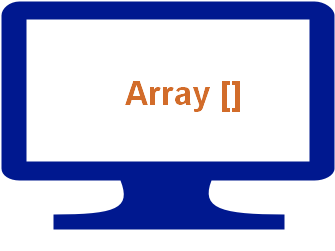# Unshift method to add element to an arrayWe can add elements at the beginning of an array by using unshift() method. Here is the general syntax for using unshift() method.
``scripts.unshift("VBScript","Perl");``
We have seen how to add element at the end of an array by using push() method.

Here scripts is our array and we are adding two new elements VBScript and Perl at the beginning of this array by using unshift() method.

Here is the complete code for unshift()
``````var scripts = new Array();
scripts = "PHP";
scripts = "ASP";
scripts = "JavaScript";
scripts = "HTML";
document.write(scripts.join(" <br> "));
document.write("<br>--Now after applying unshift()--<br>");
scripts.unshift("VBScript","Perl");
document.write(scripts.join(" <br> "));``````
The above code will add VBScript and Perl at the end of the array and display the full list. Output is here
``````PHP
ASP
JavaScript
HTML
--Now after applying unshift()--
VBScript
Perl
PHP
ASP
JavaScript
HTML``````

## unshift with key and value

Array key are re -indexed after using unshift() to add elements.
``````var scripts = new Array();
scripts = "PHP";
scripts = "ASP";
scripts = "JavaScript";
scripts = "HTML";
scripts.unshift("VBScript","Perl");
for (var key in scripts) {
document.write("key : " + key + "  =>value: " + scripts[key] + "<br>");
}``````
Output is here
``````key : 0 =>value: VBScript
key : 1 =>value: Perl
key : 2 =>value: PHP
key : 3 =>value: ASP
key : 4 =>value: JavaScript
key : 5 =>value: HTML``````## Subscribe

* indicates required
Subscribe to plus2netplus2net.com